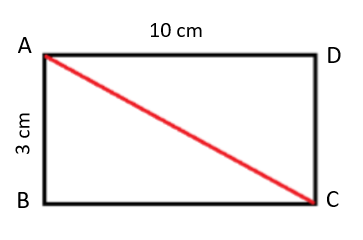ABCD is a rectangle with a length of 10 cm and a breadth of 3 cm. If AC is diagonal, then what is the area of triangle ABC?

# ABCD is a rectangle with a length of 10 cm and a breadth of 3 cm. If AC is diagonal, then what is the area of triangle ABC?1. A

25 cm2

2. B

18 cm2

3. C

15 cm2

4. D

28 cm2

Fill Out the Form for Expert Academic Guidance!l

+91

Live ClassesBooksTest SeriesSelf Learning

Verify OTP Code (required)

### Solution:

Given,

The length of a rectangle = 10 cm

The breadth of a rectangle = 3 cm

Area of triangle ABC = $\frac{1}{2}$(Area of rectangle ABCD)

$\frac{1}{2}\left(\mathrm{length}×\mathrm{breadth}\right)$

$\frac{1}{2}\left(10×3\right)$

$\frac{1}{2}×30$

= 15 cm2

Therefore, the area of triangle ABC is 15 cm2.

## Related content

 Area of Square Area of Isosceles Triangle Pythagoras Theorem Triangle Formula Perimeter of Triangle Formula Area Formulae Volume of Cone Formula Matrices and Determinants_mathematics Critical Points Solved Examples Type of relations_mathematics+91

Live ClassesBooksTest SeriesSelf Learning

Verify OTP Code (required)